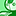# QlikView App Dev

Discussion Board for collaboration related to QlikView App Development.

Announcements
On May 18th at 10AM EDT we will answer your QlikView questions live. REGISTER
cancel
Showing results for
Did you mean:Creator II

## Percent Calculation using Max Function

Hi all,

I have a Straight Table which is having calculation like below:

Sum(UnitsSold)/max(Forecast).In the above example the calculation should be 715/252 = 2.83.

The same calculation we need to capture in Single field, as we need to use this in Set expression.

The calculated field is capturing data in row level, and summing up. And showing 5.29.

But I need 2.83 should show in the last column and it should repeat for each row.

Could anyone help me do the same.

I am attaching a sample qvw.

Thanks,

Sarif

9 RepliesSpecialist II

Hi

maybe :

Sum(total UnitsSold)/max(total Forecast)    ?

if you omit "total "  that sums each line

regardsSpecialist

Regards

PratyushCreator

Max Function use in Script - And Group by.

Use value in the front Expression.MVP

Try:

=sum( total UnitsSold)/Max(total ForecastQuantity)Creator II
Author

are you trying to say in script? or in front end.

I have a feild called[UnitsSold/ForecastQuantity].I need to use this only.

because we need to use the same field in another calculation in next chart.Creator II
Author

I already tried the same. But result is same.Creator II
Author

hi Pratyush,

Instead of creating variable, if we use max(Forecast) in denominator, then also it is working.

But i want to use the field [UnitsSold/ForecastQuantity]. As this would be using in Set expression next chart calculation.

Thanks,

SarifMVP

With the field [UnitsSold/ForecastQuantity] alone, I don't see a logic of calculating back what you are looking for. It (the possibility of solution) would be simple mathematics rather than qlik for understanding what you are trying to get.Specialist

You can probably use [UnitsSold/v_n_max_val] in your next chart.

Regards

Pratyush